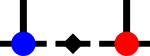SyTen## ◆ prod() [6/6]

template<Rank rank>
 SDef syten::prod ( Tensor< rank > const & a, Tensor< rank > const & b, Conj const conj = Conj::y(), SourceLocation location = SourceLocation::current() )
inline

Scalar product of two tensors.

By default, the elements of the second tensor are complex-conjugated. Legs of the tensors have to be in the same order. Equivalent to reshaping both tensors into vectors and taking the Euclidean scalar product of those.

Parameters
 a first tensor b second tensor conj if Yes (default), complex-conjugate the second tensor location Callsite identifier.Here is the call graph for this function: